﻿ 基于仿射群自调整粒子滤波泊位飞机跟踪算法 Docking Aircraft Tracking Algorithm Based on Affine Group Self-Tuning Particle Filtering

Journal of Aerospace Science and Technology
Vol. 07  No. 02 ( 2019 ), Article ID: 30849 , 6 pages
10.12677/JAST.2019.72007

Docking Aircraft Tracking Algorithm Based on Affine Group Self-Tuning Particle Filtering

Yanying Guo, Zhigang Liu

Guangzhou Civil Aviation College, Guangzhou Guangdong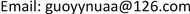Received: May 29th, 2019; accepted: Jun. 13th, 2019; published: Jun. 20th, 2019ABSTRACT

The algorithm based on affine group self-tuning particle filtering is proposed to track docking aircraft in airport, and to prevent the aircraft collision. The algorithm is mainly used to find the optimal state with minimum number of particles tracking target. The online learning estimator is used to adjust these particles, the similarity score is based on the feedback, which makes the particles move towards the optimal state. It comes to an end when all the particles are adjusted to meet the maximum degree of similarity or the maximum number of particles allowed. The algorithm achieves sparse sampling and obtains better robustness and high accuracy tracking results.

Keywords:Docking Aircraft, Tracking, Affine Group, Particle Filtering1. 引言

2. 仿射群的自调整粒子滤波算法

2.1. 仿射群

$x=\left(\begin{array}{ccc}{x}_{1}& {x}_{3}& {x}_{5}\\ {x}_{2}& {x}_{4}& {x}_{6}\\ 0& 0& 0\end{array}\right)$ (1)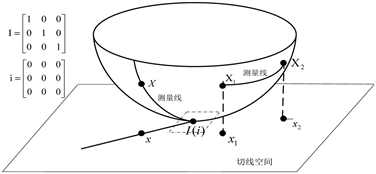Figure 1. Geometric relationship of affine groups

$\begin{array}{l}\mathrm{exp}:g\to G,X=\mathrm{exp}\left(x\right)=\sum _{i=0}^{\infty }\frac{{x}^{i}}{i!}\\ \mathrm{log}:G\to g,x=\mathrm{log}\left(X\right)=\sum _{i=1}^{\infty }\frac{{\left(-1\right)}^{i-1}}{i}{\left(I-X\right)}^{i}\end{array}$ (2)

2.2. 仿射群自调整粒子滤波算法

${X}_{t}={X}_{t-1}\cdot \mathrm{exp}\left({A}_{t-1}\right)\mathrm{exp}\left(d{\Omega }_{t}\right)$ (3)

${A}_{t-1}=a\mathrm{log}\left({X}_{t-2}^{-1}{X}_{t-1}\right)$ (4)

$\begin{array}{l}{E}_{1}=\left(\begin{array}{ccc}1& 0& 0\\ 0& 1& 0\\ 0& 0& 0\end{array}\right),\text{\hspace{0.17em}}\text{\hspace{0.17em}}{E}_{2}=\left(\begin{array}{ccc}1& 0& 0\\ 0& -1& 0\\ 0& 0& 0\end{array}\right)\\ {E}_{3}=\left(\begin{array}{ccc}0& -1& 0\\ 1& 0& 0\\ 0& 0& 0\end{array}\right),\text{\hspace{0.17em}}\text{\hspace{0.17em}}{E}_{4}=\left(\begin{array}{ccc}0& 1& 0\\ 1& 0& 0\\ 0& 0& 0\end{array}\right)\\ {E}_{5}=\left(\begin{array}{ccc}0& 0& 1\\ 0& 0& 0\\ 0& 0& 0\end{array}\right),\text{\hspace{0.17em}}\text{\hspace{0.17em}}{E}_{6}=\left(\begin{array}{ccc}0& 0& 0\\ 0& 0& 1\\ 0& 0& 0\end{array}\right)\end{array}$ (5)

${E}_{1:6}$ 测量变换节点分别是尺度、高宽比、旋转、斜交角度、x变换和y变换  。

1) 通过(6)式 ${\stackrel{^}{X}}_{t}$${\stackrel{^}{X}}_{t-1}$ 计算速率 ${A}_{t-1}$

2) 通过状态变换(5)式传递 ${\chi }_{t}$ 到新的状态 ${\chi }_{t}^{0}={\left\{{X}_{t,i}^{0}\right\}}_{i=1}^{\Delta {N}_{0}}$

3) 调整 ${\chi }_{t}^{0}$ 到最优状态 ${\stackrel{^}{\chi }}_{t}^{0}={\left\{{\stackrel{^}{\chi }}_{t:i}^{0}\right\}}_{i=1}^{\Delta {N}_{0}}$ 通过自调整算法，然后计算 ${S}_{\mathrm{max}}^{0}=\mathrm{max}{\left\{Sim\left(o\left({\stackrel{˜}{X}}_{t:i}^{0}\right)\right)\right\}}_{i-1}^{\Delta {N}_{0}}$

4) $n=\Delta {N}_{0}$$k=0$

5) 循环：当 ${S}_{\mathrm{max}}^{k}<{\mu }_{T}-{\sigma }_{T}$$n<{N}_{total}$ 完成以下步骤：

6) a) $k=k+1$ ；b) $\Delta {N}_{k}=\mathrm{min}\left\{{\alpha }_{inc}\Delta {N}_{k-1},{N}_{total}-n\right\}$ ；c) 通过 ${\stackrel{˜}{X}}_{t}^{k-1}$ 重采样产生新的粒子集 $\Delta {\chi }_{t}^{k}={\left\{{X}_{t,j}^{k}\right\}}_{j-1}^{\Delta {N}_{k}}$ ；d) 传播新的粒子：用 $d{\Omega }_{t,j}^{k}~{N}_{g}$ 传播 ${X}_{t,j}^{k}={X}_{t,j}^{k}\mathrm{exp}\left(d{\Omega }_{t,j}^{k}\right)$ ；e) 调整 $\Delta {\chi }_{t}^{k}$ 到它们邻近的最优状态 $\Delta {\stackrel{˜}{\chi }}_{t}^{k}$ ；f) $n=n+\Delta {N}_{k}$ ；g) 传播所有调整粒子： ${\stackrel{˜}{\chi }}_{t}^{k}=\left\{{\stackrel{˜}{\chi }}_{t}^{k-1},\Delta {\stackrel{˜}{\chi }}_{t}^{k}\right\}={\left\{{\stackrel{˜}{X}}_{t,i}^{k}\right\}}_{i=1}^{n}$ ；h) ${S}_{\mathrm{max}}^{k}=\mathrm{max}{\left\{Sim\left(o\left({X}_{t,i}^{k}\right)\right)\right\}}_{i-1}^{n}$

7) 计算 ${\stackrel{^}{X}}_{t}={{\stackrel{˜}{X}}_{t,i}^{k}|}_{Sim\left(o\left({\stackrel{˜}{X}}_{t,i}^{k}\right)\right)-{S}_{\mathrm{max}}^{k}}$${\stackrel{^}{S}}_{t}={S}_{\mathrm{max}}^{k}$

8) 条件更新：假如 ${\stackrel{^}{S}}_{t}>{\mu }_{B}+{\sigma }_{B}$ 那么利用 ${\stackrel{^}{X}}_{t}$ 更新观测模型；

9) 通过 ${\stackrel{˜}{\chi }}_{t}^{k}$ 的重采样产生下一帧 ${\chi }_{t}={\left\{{X}_{t,i}\right\}}_{i=1}^{\Delta {N}_{0}}$

3. 泊位飞机跟踪实验结果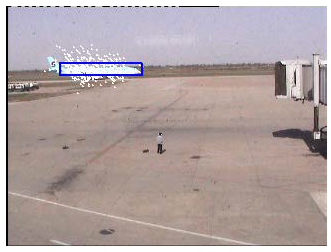(a) 第10帧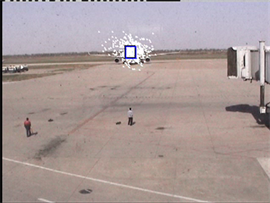(b) 第253帧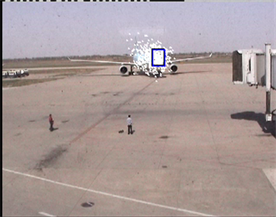(c) 第332帧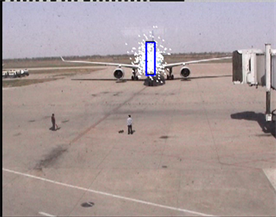(d) 第483帧

Figure 2. Tracking effect image of PF algorithm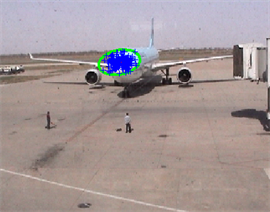(a) 第575帧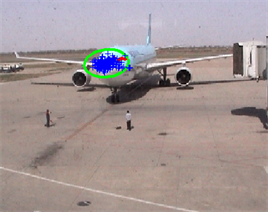(b) 第703帧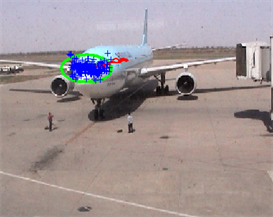(c) 第932帧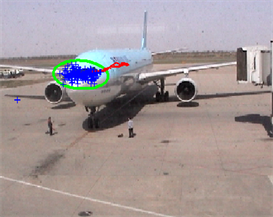(d) 第1205帧

Figure 3. The tracking effect image of the algorithm of this paper

4. 结论

Docking Aircraft Tracking Algorithm Based on Affine Group Self-Tuning Particle Filtering[J]. 国际航空航天科学, 2019, 07(02): 57-62. https://doi.org/10.12677/JAST.2019.72007

1. 1. Yilmaz, A., Javed, O. and Shah, M. (2006) Object Tracking: A Survey. ACM Computing Surveys, 38, 1-45. https://doi.org/10.1145/1177352.1177355

2. 2. Nummiaroa, E.K.-M.K. and Gool, L. (2003) An Adaptive Color-Based Particle Filter. Image and Vision Computing, 21, 99-110. https://doi.org/10.1016/S0262-8856(02)00129-4

3. 3. 李培华. 一种新颖的基于颜色信息的粒子滤波器跟踪算法[J]. 计算机学报, 2009, 32(12): 2455-2463.

4. 4. Pocok, J., Dance, S. and Lawless, A. (2011) State Estimation Using the Particle Filter with Mode Tracking. Computer Fluids, 46, 392-397. https://doi.org/10.1016/j.compfluid.2011.02.009

5. 5. 李远征, 卢朝阳, 高全学, 李静. 基于多特征融合的均值迁移粒子滤波跟踪算法[J]. 电子与信息学报, 2010, 32(2): 411-415.

6. 6. 郑华, 谭博, 裴承鸣. 粒子滤波算法在多传感器测量中的应用[J]. 现代电子技术, 2014, 37(1): 25-30.

7. 7. Wang, J.Y., Chen, X.L. and Gao, W. (2005) Online Selection Discriminative Tracking Features Using Particle Filter. In: IEEE Conference on Computer Vision and Pattern Recognition, IEEE Press, New York, 1037-1042.

8. 8. 林霖. 基于几何特征的飞机部位识别跟踪算法[J]. 红外, 2007, 28(8): 131-135.

9. 9. Porikli, F., Tuzel, O. and Meer, P. (2006) Covariance Tracking Using Model Update Based on Lie Algebra. Proceedings of the IEEE Computer Society Con-ference on Computer Vision and Pattern Recognition, New York, 17-22 June 2006, 728-735.

10. 10. Li, M., Tan, T., Chen, W. and Huang, K. (2012) Efficient Object Tracking by Incremental Self-Tuning Particle Filtering on the Affine Group. IEEE Transactions on Image Processing, 21, 1298-1313. https://doi.org/10.1109/TIP.2011.2169970